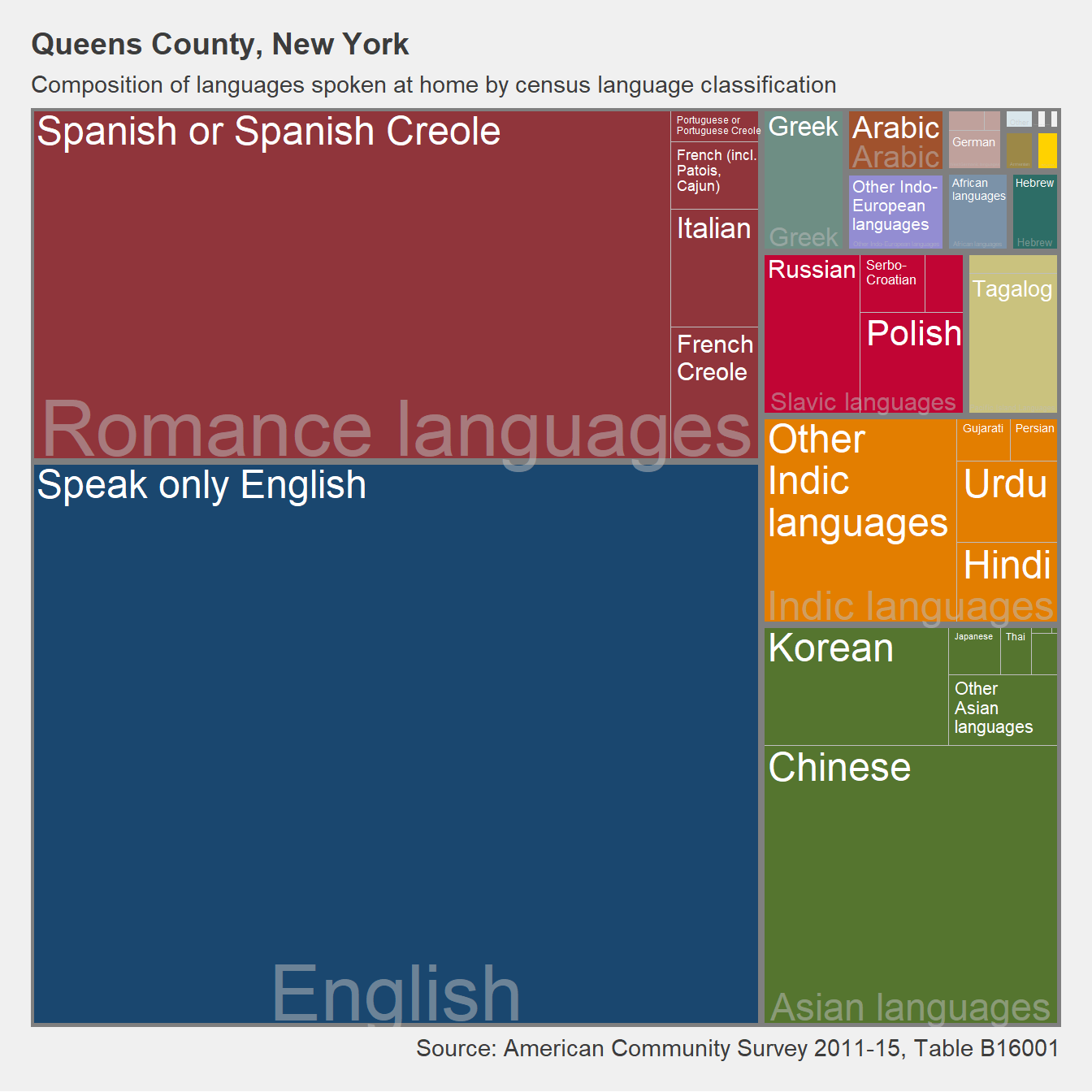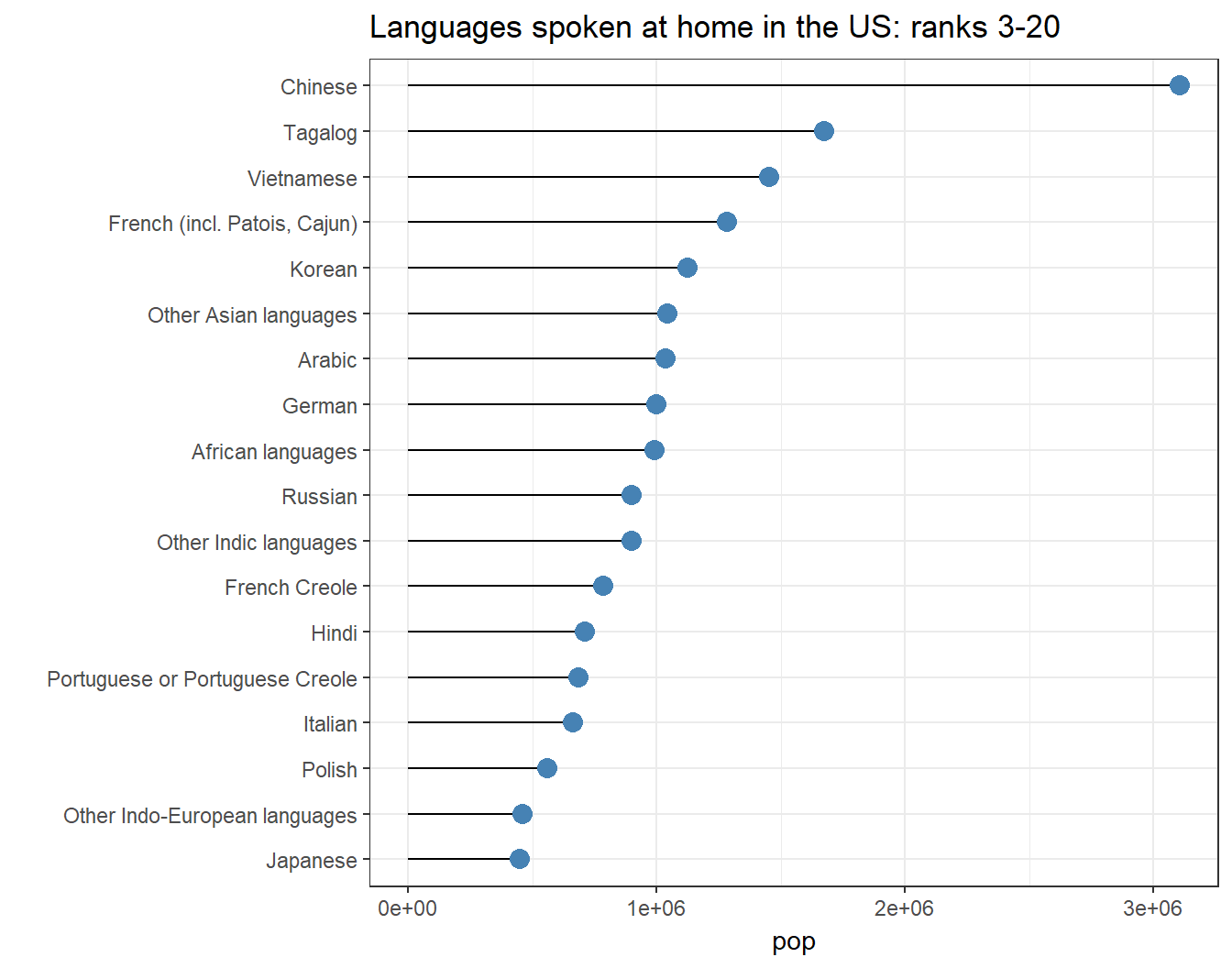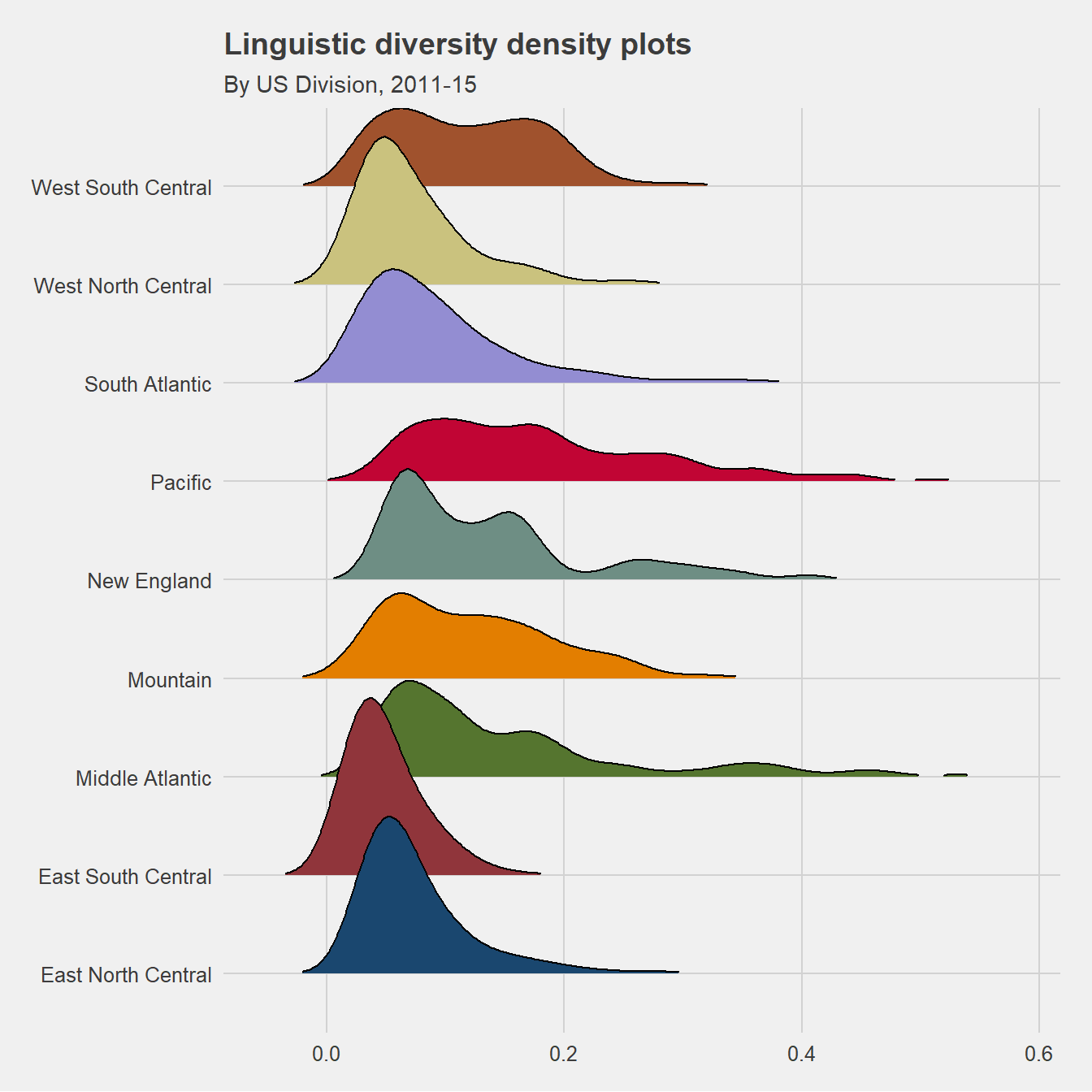# locating linguistic diversity in the usa

This post investigates linguistic diversity in the United States utilizing data made available by the US Census. We consider census language classifications, and introduce a simple methodology for quantifying linguistic diversity using entropy scores.

The post is largely exploratory, and a bit of an excuse to play with some different data visualization packages, including ggridges and treemapify, and to see about America some.

library(tidycensus)
options(tigris_use_cache = TRUE)
library(tidyverse)
library(sf)
library(DT)

## Language data and the census

Language data in the US census live in census table B16001, which provides summary counts of speakers by language spoken at home. The load_variables function of the tidycensus package allows for nice and easy interaction with census tables and their constituent variables; using this function, then, we gather the relevant language variables included in table B16001,

langs <- load_variables(2015, "acs5", cache = FALSE) %>%
filter(grepl("B16001",name) &
!grepl("Margin|Speak English|Total$",label)) %>% mutate(name=gsub("E","",name), label=gsub("^.*!!","",label))%>% select(-concept) The US census provides counts of speakers for 40 languages/language categories; these categories are cleaned up some in the table below, where we add some details implicit in the census’ classification scheme. While the classifications would leave a linguistic typologist nonplussed, for our purposes here they are fine. Using tidycensus, we pull counts of speakers for the languages listed above for each county in the US, along with the summary_var (or denominator), which amounts to the population over 5. langData <- tidycensus::get_acs( geography = "county", variables = langs$name,
summary_var="B16001_001",
survey="acs5",
year=2015) %>%
left_join(sumLangs,by=c("variable"="name"))

As an example to explore the data some, we consider languages spoken at home for a county famous for its linguistic diversity: Queens County, New York. Of the 40 language classifications included in the census, Queens is home to speakers of 39 (per 2011-15 5-year estimates).

Using the treemapify package, the plot below summarizes languages spoken in Queens. Rectangle areas represent the proportion of total speakers, colors reflect language types/families, and sub-tiles reflect within-type languages. While only exploratory, the plot nicely captures how diverse Queens is in terms of languages spoken by its residents.

library(treemapify)
library(ggthemes)

langData%>%
filter(NAME=='Queens County, New York')%>%
ggplot(aes(area = estimate,
fill = reorder(type,-estimate),
label = label,
subgroup = type))+
geom_treemap()+
geom_treemap_subgroup_border() +
geom_treemap_subgroup_text(place = "bottom",
grow = F,
alpha = 0.5,
colour ="gray",
min.size = 0)+
geom_treemap_text(colour = "white",
place = "topleft",
reflow = T)+
scale_fill_stata()+
theme_fivethirtyeight()+
theme(legend.position = "none",
plot.title = element_text(size=14))+
labs(title = "Queens County, New York",
subtitle = "Composition of languages spoken at home by census language classification",
caption="Source: American Community Survey 2011-15, Table B16001")## Languages in the US

Next, we take a quick look at languages with the largest speaker-bases in the US. While English and Spanish clearly rank first and second, respectively, the plot below illustrates the languages with the eighteen next largest speaker-bases.

langData %>%
data.frame()%>%
group_by(label)%>%
summarise(pop=sum(estimate))%>%
arrange(desc(pop))%>%
slice(3:20)%>%
ggplot(aes(x=label, y=pop)) +
geom_segment(aes(x=reorder(label,pop),
xend=label,
y=0, yend=pop)) +
xlab("")+
geom_point(size=3.5,
color='steelblue') +
labs(title="Languages spoken at home in the US: ranks 3-20") +
coord_flip()+
theme_bw()## Linguistic diversity as entropy

To get a better sense of where the linguistic diversity attested in the plot above distributes geographically, we calculate entropy scores for each county within the US. Entropy, $E$, for a given county, $i$, is calculated as $E_i = \sum_{r=1}^n Q_r ln\frac{1}{Q_r}$ where $Q_r$ is the proportion of speakers of language $r$, and $n$ is the total number of languages spoken (also know as Richness).

Per this metric, maximum entropy would mean that each of the 4o census language types were spoken by equal numbers of people within a given county; minimum entropy would mean that all speakers within a given county spoke the same language .

This metric (and like-minded variants) is often used in socio-demographic research to assess racial/ethnic diversity, as well as in ecological research to evaluate species diversity and richness.

byCounty <- langData %>%
data.frame%>%
mutate(prop = estimate/summary_est)%>%
mutate(eIndex = prop *  log(1/(prop)) )%>%
filter(!is.na(eIndex)& grepl("Hawaii$|Alaska$",NAME)==FALSE)%>%
group_by(GEOID,NAME)%>%
summarize(entropy=sum(eIndex))%>%
mutate(entropy=round(entropy/log(40),3))%>%
#scale to max entropy
ungroup()

## Locating linguistic diversity

Based on these derived entropy scores, the table below summarizes the fifty most linguistically diverse counties in the US. As can be noted, Queens tops the list. A close second is Santa Clara County, California, which is home to Silicon Valley. Alameda and San Mateo counties are part of the SF Bay Area. As such, it would seem that entropy scores align well with intuition.

byCounty%>%
data.frame()%>%
arrange(desc(entropy))%>%
slice(1:50)%>%
select(NAME,entropy)%>%
DT::datatable(selection="none",
class = 'cell-border stripe',
rownames = FALSE,width="100%",
escape=FALSE)%>%
DT::formatStyle('entropy',
background = DT::styleColorBar(byCounty$entropy, 'steelblue'), backgroundSize = '80% 70%', backgroundRepeat = 'no-repeat', backgroundPosition = 'right') %>% DT::formatStyle(c(1:2),fontSize = '85%') For a more comprehensive perspective on this geospatial variation, we plot entropy scores for the entire US by county using the leaflet package. Using the tigris package, we first pull US county and division shapefiles, the latter to provide a more macro-geospatial lens on variation. library(rmapshaper) library(tigris) options(tigris_class = "sf") cts <- tigris::counties(cb=TRUE)%>% select(GEOID,geometry) %>% rmapshaper::ms_simplify() divs <- tigris::divisions(cb=TRUE)%>% st_transform(crs = "+init=epsg:4326") Divisions include: ##  "New England" "Middle Atlantic" "East North Central" ##  "West North Central" "South Atlantic" "Mountain" ##  "Pacific" "East South Central" "West South Central" Call to leaflet: library(leaflet) library(widgetframe) pal <- colorNumeric(palette = "RdPu", domain = byCounty$entropy)

x <- byCounty %>%
left_join(cts)%>%
st_as_sf()%>%
st_transform(crs = "+init=epsg:4326")%>%
leaflet(width="100%",height='400') %>%
setView(lng = -98.35, lat = 39.5, zoom = 4) %>%
addProviderTiles ("CartoDB.Positron",
options = providerTileOptions(minZoom = 4, maxZoom = 7))%>%
addPolygons(popup = ~ NAME,
fill = TRUE,
stroke = TRUE,weight=1,
fillOpacity = 1,
color="white",
fillColor=~pal(entropy))%>%
addPolygons(data=divs,
fill=FALSE,
color="gray",
stroke = TRUE,
weight=1)%>%
addCircleMarkers(data=st_centroid(divs),
radius=0,
label=~NAME,
labelOptions = labelOptions(noHide = T,
textOnly = TRUE,
offset=c(-25,-10)))%>%
addLegend("bottomright",
pal = pal,
values = ~ entropy,
title = "Entropy",
opacity = 1)
frameWidget(x)

Perhaps unsurprisingly, linguistic diversity as approximated by entropy scores is highest in major metropolitan areas in the northeast, southern Florida, the southwest, Bay Area & southern California, and the Pacific Northwest.

Lastly, we consider the distribution of county entropy scores within each division. The ggridges package makes this task relatively straightforward. The figure below, then, illustrates density plots for county-level entropy scores by division.

While the plot is comprised of the same data as the choropleth map above, I think it provides a more succinct perspective on variation in linguistic diversity across divisions, as well as a nice ‘profile’ of linguistic diversity within divisions.

#devtools::install_github("jaytimm/geodatr")
library(geodatr)
library(ggridges)

byCounty %>%
mutate(State=as.character(gsub("^.+, ","",NAME)))%>%
left_join((geodatr::us_regions)) %>%
na.omit()%>%
ggplot(aes(entropy, Div_Name, fill = Div_Name)) +
scale_fill_stata()+
theme_fivethirtyeight()+
geom_density_ridges(rel_min_height = 0.01) +
theme(legend.position = "none",
plot.title = element_text(size=14)) +
ylab("")+
labs(title = "Linguistic diversity density plots",
subtitle = "By US Division, 2011-15")## FIN

So, some different perspectives on linguistic diversity in the United States via US Census (ACS) data, and some different approaches to visualizing variation across distributions. Despite the focus on linguistic diversity in this post, the US is still very much a monolingual, English speaking country in the aggregate.

langData%>%
filter(label=='Speak only English') %>%
summarise_at(vars(estimate,summary_est),sum)%>%
mutate(perEnglishOnly=round(estimate/summary_est,3)*100)
Share